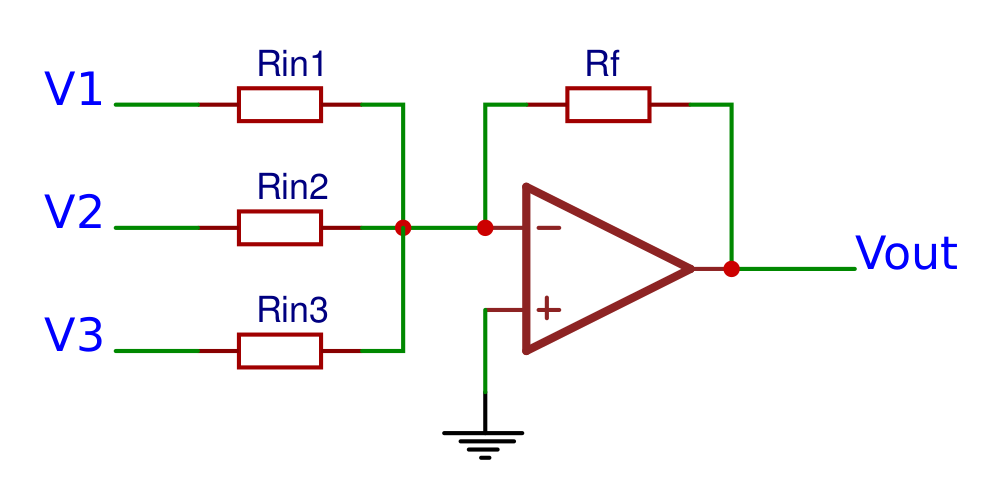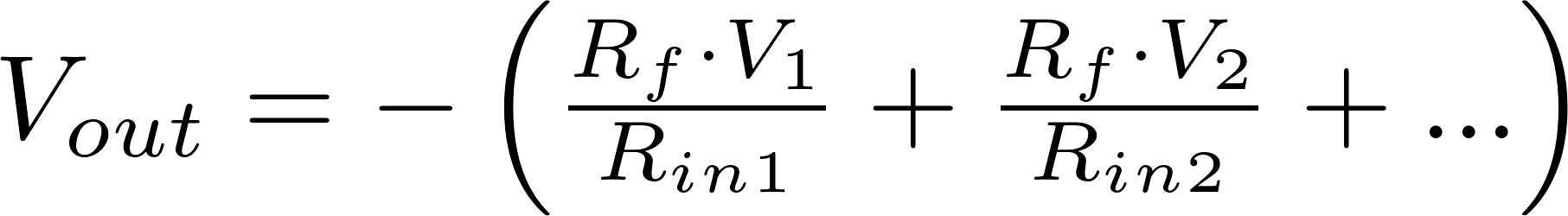# Inverting summing amplifier

The inverting summing opamp works the same as the inverting opamp, so the input terminals are inverted and the input voltage is connected to the negative terminal and the positive terminal is connected to ground, but there are multiple input voltages connected to the negative terminal instead of only one.### FormulaV in is the input voltage and is measured in volt (V)
V out is the output voltage and is measured in volt (V)
R is the symbol for resistance and is measured in ohm (Ω).
A is the symbol for gain.